• /*  快速排序  基本思想  选定每次排序的基准数据 在剩下的位置将小于基准值的数据放在... 递归实现Quick_Sort1(int *arr,int len):快排一次划分的时间复杂度为O(logn) 最坏就是在有序条件下 时间复杂度为O(n^2...
/*  快速排序  基本思想    选定每次排序的基准数据 在剩下的位置将小于基准值的数据放在基准值得左边，大于基准值的数据放到基准值的右边    一次划分之后 如果此基准值的左右两边仍存在大于两个数据  则继续划分排序 直至每个数字都有序   递归实现Quick_Sort1(int *arr,int len):快排一次划分的时间复杂度为O(logn) 最坏就是在有序条件下 时间复杂度为O(n^2)   整体时间复杂度为O(n*logn)   非递归实现Quick_Sort2(int *arr,int len)
快排三种实现方式    第一种就是选取第一个元素或者最后一个元素作为基准 代码是Quick_Sort1 和Quick_Sort2    这样的坏处是如果所给序列是有许多的  那么时间复杂度太大 选取的基准点就失去了意义 并不能称作是基准值了    第二种随机取值法     绝大多数情况下保证了时间复杂度最好情况是O(logn)  但是最坏情况下 假如整个数组的数字都相等 那么时间复杂度还是能达到O(n^2)    第三种 三数取中    找到low high  mid = low + ((high - low) >> 1);//计算数组中间的元素的下标
取这三个下标对应的最小值 保证arr[low]是这三个数之间的第二大值 然后又可以和普通划分函数一样
*/
void swap(int *a,int *b)
{
int tmp  = *a;
*a = *b;
*b = tmp;
}
//第一种划分函数:以第一个数字为基准值进行一次划分 最后返回基准的下标
int partion(int *arr,int low,int high)
{
if(arr == NULL )
return -1;
int tmp = arr[low];

while(low < high)
{
while((low < high) && arr[high] >= tmp)
{
high--;
}
if(low == high)//如果遇到比基准值小的 放到low的位置
{
break;
}
else
{
arr[low] = arr[high];
}

while((low < high) && arr[low] <=  tmp)
{
low++;
}
if(low == high)
{
break;
}
else
{
arr[high] = arr[low];
}
}
arr[low] = tmp;
return low;
}
//第二种划分函数:随机选取基准划分
int part(int *arr,int low,int high)
{
srand((unsigned)time(NULL));
//产生随机位置
int pivotPos = rand()%(high - low) + low;
//将此随机位置的值与low位置的值交换 又可以和普通划分函数一样
swap(arr[pivotPos],arr[low]);

int tmp = arr[low];

while(low < high)
{
while((low < high) && arr[high] >= tmp )
{
high--;
}
if(low == high)//如果遇到比基准值小的 放到low的位置
{
break;
}
else
{
arr[low] = arr[high];
}

while((low < high) && arr[low] <=  tmp)
{
low++;
}
if(low == high)
{
break;
}
else
{
arr[high] = arr[low];
}
}
arr[low] = tmp;
return low;
}
//第三种划分函数
int Select_Mid(int *arr,int low,int high)
{
//int mid = low + ((high - low) >> 1);//计算数组中间的元素的下标
int mid = low + ((high - low) >> 1);
//使用三数取中法选择枢轴
if (arr[mid] > arr[high])//目标: arr[mid] <= arr[high]
{
swap(arr[mid],arr[high]);
}
if (arr[low] > arr[high])//目标: arr[low] <= arr[high]
{
swap(arr[low],arr[high]);
}
if (arr[mid] > arr[low]) //目标: arr[low] >= arr[mid]
{
swap(arr[mid],arr[low]);
}
return arr[low];
}
//三数取中的一次划分
int part1(int *arr,int low,int high)
{

int tmp = Select_Mid(arr,low,high);

while(low < high)
{
while((low < high) && arr[high] >= tmp )
{
high--;
}
if(low == high)//如果遇到比基准值小的 放到low的位置
{
break;
}
else
{
arr[low] = arr[high];
}

while((low < high) && arr[low] <=  tmp)
{
low++;
}
if(low == high)
{
break;
}
else
{
arr[high] = arr[low];
}
}
arr[low] = tmp;
return low;
}
static void Quick(int *arr,int start,int end)
{
int par = part1(arr,start,end);
if(par > start + 1)
{
Quick(arr,start,par-1);
}
if(par < end -1)
{
Quick(arr,par+1,end);
}
}
void Quick_Sort1(int *arr,int len)
{
if(arr == NULL ||len < 0)
return;
for(int i = 0 ;i < len - 1 ;++i)
{
Quick(arr,0,len-1);
}
}
快排非递归实现
//用栈保存每一次的一对low和high区间 空间复杂度为O(n*logn)
void Quick_Sort2(int *arr,int len)
{
if( arr == NULL ||len < 0)
return ;
int low = 0;
int high = len - 1;
int par =  partion(arr,low,high);
stack<int> st;
if(par > low + 1)
{
st.push(low);
st.push(par-1);
}
if(par < high - 1)
{
st.push(par+1);
st.push(high);
}
while(!st.empty())
{
int high = st.top();st.pop();
int low = st.top();st.pop();
int par = partion(arr,low,high);
if(par > low + 1)
{
st.push(low);
st.push(par-1);
}
if(par < high - 1)
{
st.push(par+1);
st.push(high);
}
}
}
/*
快排优化一
在low和high之间的举例为10以内时，可以选择直接插入排序 节省时间
*/
//快排优化一 在划分到适合的长度进行直接插入排序
void insert_sort(int *arr,int low,int high)
{
if(arr == NULL || low < 0 || high < 0)
return ;
int tmp = 0;
int j;
for(int i = low+1;i < high; i++)
{
tmp = arr[i];
for(j = i-1;j >= 0;j--)
{
if(arr[j] > tmp)
{
arr[j+1] = arr[j];
}
else
{
break;
}
}
arr[j+1] = tmp;
}
}
static void Quick1(int *arr,int start,int end)
{
int par = part1(arr,start,end);
if(end - start + 1 < 10)
{
insert_sort(arr,start,end);
}
else
{
if(par > start + 1)
{
Quick(arr,start,par-1);
}
if(par < end -1)
{
Quick(arr,par+1,end);
}
}
}
void Quick_Sort3(int *arr,int len)
{
if(arr == NULL ||len < 0)
return;
for(int i = 0 ;i < len - 1 ;++i)
{
Quick(arr,0,len-1);
}
}
/*
快排优化二    在一次划分之后 将与基准值相等的元素聚集在一起不再进行下一次的划分操作 可以减少迭代查找次数    具体操作将与基准值相等的数放到数组的两端 一次划分完成后 将这些数字挪回基准值得范围 具体过程如下图所示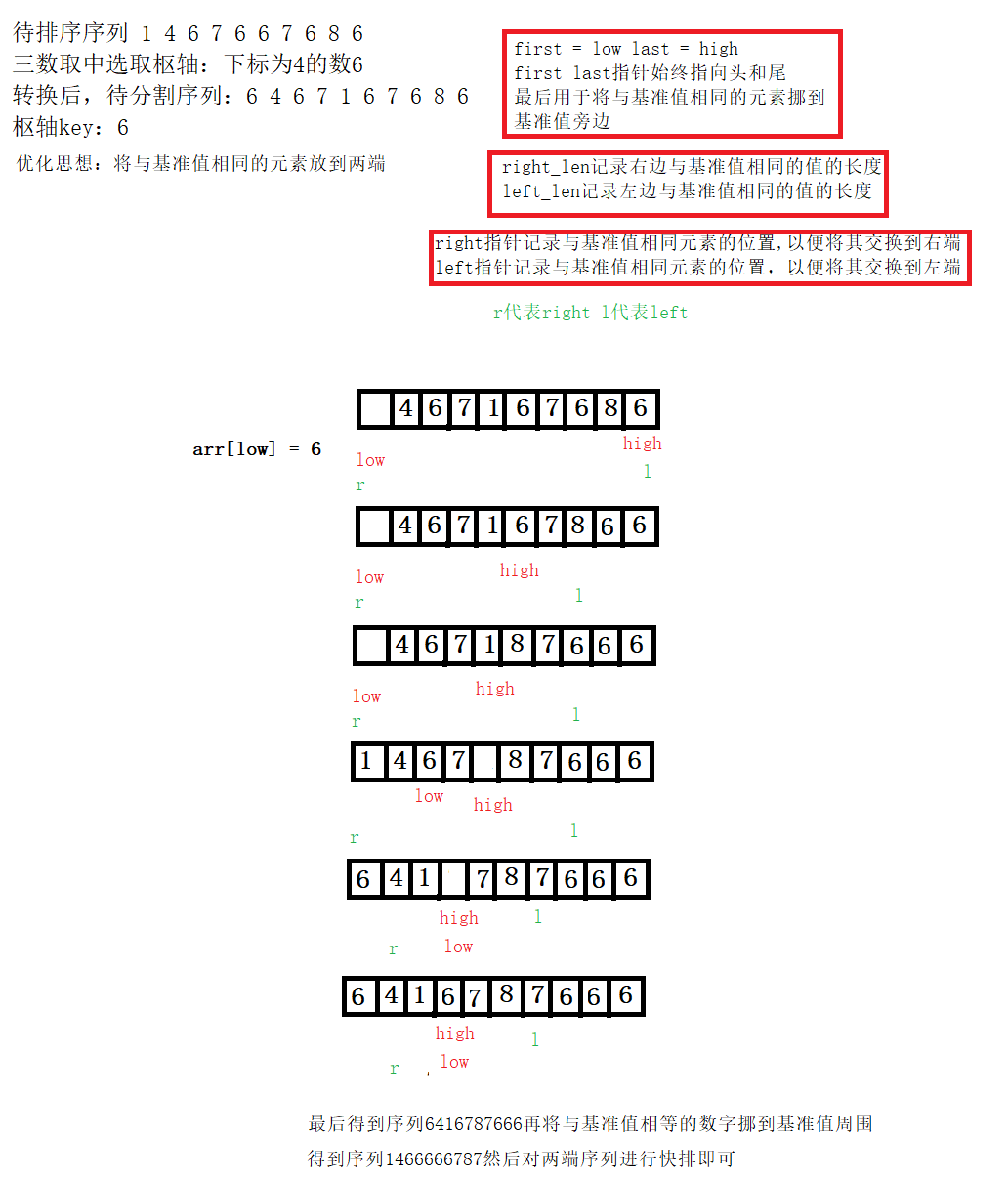*/
//快排优化二 一次划分后将基准值调至靠近基准值周围 与基准值相等的数字不再参加下一次划分
void QuickSort(int *arr,int low,int high)
{
int first = low;
int last = high;

int left = low;
int right = high;

int leftLen = 0;
int rightLen = 0;

//一次分割
int key = Select_Mid(arr,low,high);//使用三数取中法选择枢轴

while(low < high)
{
while(high > low && arr[high] >= key)
{
if (arr[high] == key)//处理相等元素
{
swap(&arr[right],&arr[high]);
right--;
rightLen++;
}
high--;
}
arr[low] = arr[high];

while(high > low && arr[low] <= key)
{
if (arr[low] == key)
{
swap(&arr[left],&arr[low]);
left++;
leftLen++;
}
low++;
}
arr[high] = arr[low];
}
arr[low] = key;

//一次快排结束
//把与枢轴key相同的元素移到枢轴最终位置周围
int i = low - 1;
int j = first;
while(j < left && arr[i] != key)
{
swap(&arr[i],&arr[j]);
i--;
j++;
}
i = low + 1;
j = last;
while(j > right && arr[i] != key)
{
swap(&arr[i],&arr[j]);
i++;
j--;
}
//如果左右两端都还有超过两个数据 那么就需要进行划分排序
if(low-1 > first+1)
{
QuickSort(arr,first,low - 1 - leftLen);
}
if(low+1 < last-1)
{
QuickSort(arr,low + 1 + rightLen,last);
}

}

展开全文• ## 快排优化Python表示

千次阅读 2016-03-18 14:54:54
基本快速排序分析 以从小到大排序为例 * 选取一个主元（选取方式多样） ...快排 1.3990s limit exceed limit exceed limit exceed 优化 0.6570s 0.9410s 0.9900s 0.0699s
基本快速排序分析

以从小到大排序为例  * 选取一个主元（选取方式多样）  * 利用主元，将序列分为两个子序列，左侧都比主元小，右侧都比主元大。  * 对两个子序列重复此操作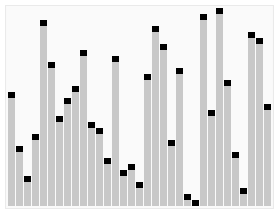例如取第一个元素,代码表示如下：
def qsort(arr):
if len(arr) <= 1:
return arr
else:
pivot = arr
return qsort([x for x in arr[1:] if x < pivot]) + \
[pivot] + \
qsort([x for x in arr[1:] if x >= pivot])

优化方案
主元的选取  上面的算法有很大的问题，对于升序或降序序列，效率很差，我优化后的方式是主元取序列首中尾  三个值取平均数，网上有些取三个值的中值的，我认为没必要，为了效率还要将中值换到首或尾。序列中可能有一些和主元相等的元素，上面直接将其并入子序列中了，最好是将其和主元聚集  在一起，子序列缩减幅度也会更快
这样的话定义一个函数：
def getMidNum(list):
return (list + list[len(list) - 1] + list[len(list)/2])/3
def qsort(arr):
if len(arr) <= 1:
return arr
else:
pivot = getMidNum(arr)
return qsort([x for x in arr[0:] if x < pivot]) + \
[x for x in arr[0:] if x == pivot] + \
qsort([x for x in arr[0:] if x > pivot])


对比
分别构造长度为10000的随机数列表，升序列表，将序列表和等值列表，对比二者的表现
方法\序列随机升序降序等值快排1.3990slimit exceedlimit exceedlimit exceed优化0.6570s0.9410s0.9900s0.0699s
展开全文• 对给定的图结构，主体利用贪心算法实现求解最小生成树的Kruskal算法，其中每次查找权值最小的边用快速排序实现优化。每次在满足和已选边不构成回路的条件下选择一条权植最小的边，添加到新的生成树中。
• ## 快排总结和优化

千次阅读 2017-10-08 10:43:24
快排总结和优化emmm……把以后可能用到的快排优化后的快排整理了一下。
快排总结和优化

emmm……把以后可能用到的快排和优化后的快排整理了一下。
快速排序是一种分治的算法。  与归并排序不同，归并排序是先对子数组分别排序，再归并使整个数组有序；  快速排序是不断地递归对数组进行切分，当子数组有序时整个数组也就自然有序了。
1.基本算法
基于二分法的快排，左右分别递归：
public static void quickSort(int[] array, int lo, int hi) {
if(hi <= lo ) return;
int pivot = partition(array, lo, hi);
quickSort(array, lo, pivot - 1);
quickSort(array, pivot + 1, hi);
}


2.切分算法

快速排序的关键在于切分算法，切分算法有多种写法。

快速切分原理图 ：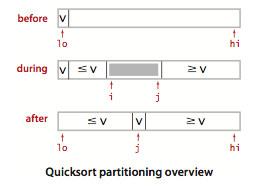I.切分一：

原理如图，一般策略是随意选取a[lo]作为轴点v（切分元素）； 然后从左往右扫描直到找到一个大于它的元素，再从右往左扫描知道找到一个小于等于它的元素； 如此继续，直到最后i,j两个指针相遇时，再将选取的轴点v和左子数组最右侧的元素a[j]交换，然后返回j(或i)即可。

private static int partition(int[] array, int lo, int hi) {
int i = lo, j = hi + 1;
int pivot = array[lo];//以首元素作为候选轴点
while(true) {
//从左往右直到找到大于或等于pivot的元素结束
while(array[++i] < pivot) if(i == hi) break;
//从右往左直到找到小于或等于pivot的元素结束
while(pivot < array[--j]) if(j == lo) break;
if(i >= j) break;
//交换找到的两个元素
swap(array, i, j);
}
//最后将轴点的值与i,j相遇的位置的值进行交换
swap(array, lo, j);
return j; //此时找到轴点位置j
}

注：也可以先从右往左扫描； 这个算法中break用来检测越界，要是越界就用break退出。

II.切分二：

第二个版本（初学时写的比较多）先从右往左扫描，并且减少了swap()额外空间带来的开销，但也有些细节需要注意。

private static int partition(int[] list, int lo, int hi) {
int pivot = list[lo];
while(lo < hi){
//从右往左找到小于或等于pivot的元素，h向左拓展
while(lo < hi && pivot < list[hi]) hi--;
if(lo < hi){
list[lo++] = list[hi];
}
//从左往右找到大于pivot的元素，lo右拓展
while(lo < hi && list[lo] < pivot) lo++;
if(lo < hi){
list[hi--] = list[lo];
}
}
list[lo] = pivot;
return lo;
}

这里面加if语句是为了，防止lo大于hi时hi（lo）继续拓展，如果不加这句,它的上一句改成pivot<=list[hi],它的下一句需要去掉++，之所以这样写是为了减少防止有大量重复元素时，由于过多的拓展，时间复杂度退化为O(

n2
$n^2$)，但同时也增加了交换次数，使快排的稳定性更难保持，这是一个小细节。

3.基本快排的补充
原地切分：使用一个辅助数组来辅助，但切分后的数组复制回去的开销会大很多。保持随机性：快排是依赖输入的算法，快排的开始前可以写一个shffle()方法将数组打乱,或直接可以写:
Random rnd = new Random();
int index = rnd.nextInt(array.length);
swap(array, pivot, index);
开始时用来随机选取轴点，避免快排对输入的依赖。
避免等值元素的交换：实现快排时要做到这点，不然时间复杂度会退化到O(

n2
$n^2$)。递归的终止：实现快排的常见错误是不能将轴点放入正确位置，就会导致程序在轴点正好是子数组的最大或最小元素时陷入无限循环。

4.性能特点

对于长度为n的无重复数组，快排平均需要~

2nlnn
$2nlnn$（

1.39nlogn
$1.39nlogn$）次比较，以及

1/6
$1/6$次交换；  快排最多需要

n2/2
$n^2/2$次比较，但随机打乱数组能避免这种情况。

5.快排的改进
I.小数组切换到插入排序
对小数组快速排序比插入排序慢，还有递归调用，因此以在快排的过程中遇到小数组时应该切换到插入排序。

将quickSort()中的语句：if(hi <= lo) return;  替换为：if(hi <= lo + M) { insertionSort(a, lo, hi); return; }  转换参数M的最佳值与系统相关，但在5~15之间的任意值在大多数情况下都能令人满意。

II.三向切分快排

对于有大量重复元素的数组，这种方法比标准的快速排序的效率要高很多

三向切分快排原理图：三向切分快排思路跟普通快排差不多，只是把轴点变成了“轴线”，“轴线”部分的值就是轴点值，大的元素放到轴点值右边，小的元素放在轴点值左边。  实现时，每次都让lt下标的值等于轴点值，用i扫秒lt右侧，碰到与轴点相等的值就继续向后扫描，这样就使得中间部分的元素值都等于轴点值了。也就是说工作指针 i 经过的地方都等于轴点值。  切分结束的条件也跟普通快排差不多，当i的位置大于gt的位置就结束算法。  跟普通快排一样是小值扔轴点左边，大值扔轴点右边。
public static void quick3waySort(int[] array, int lo, int hi) {
if(hi <= lo) return;
int lt = lo, i = lo + 1, gt = hi;
int pivot = array[lo];//轴点取最低位
//一次切分
while(i <= gt) {
//i处的值小于轴点就与lt交换，并继续向后扫描，同时lt也右移
if      (array[i] < pivot) swap(array, lt++, i++);
//i处的值大于轴点就和gt交换，然后gt左移
else if (array[i] > pivot) swap(array, i, gt--);
//碰到与轴点相等的值继续向后扫描
else                       i++;
}
quick3waySort(array, lo, lt - 1);
quick3waySort(array, gt + 1, hi);
}


III.双轴点快排
还有一种和三向切分快排类似的双轴快排，Arrays.sort()就主要是用它来实现的。  与三向切分快排不同的是，双轴快排的两个轴分别为lo, hi。  在双轴快排中部即工作指针 i 扫描过的地方比左轴点的值大，比右轴点的值小，但仍然无序。
  private static void dualpivotQuickSort(int[] array, int lo, int hi) {
if (hi <= lo) return;

//开始划分前确保a[lo] <= a[hi]
if (array[hi] < array[lo]) swap(array, lo, hi);

int lt = lo + 1, gt = hi - 1;
int i = lo + 1;
//一次切分
while (i <= gt) {
//i处的值小于左轴点就与lt交换，并继续向后扫描，同时lt也右移
if       (array[i] < array[lo]) swap(array, lt++, i++);
//i处的值大于右轴点就和gt交换，然后gt左移
else if  (array[hi] < array[i]) swap(array, i, gt--);
else                    i++;
}
//一次切分结束后在序列中间得到一个小值和大值，让他们分别和两轴点交换
swap(array, lo, --lt);
swap(array, hi, ++gt);

dualpivotQuickSort(array, lo, lt-1);
//如果中部序列低位的值小于高位的值，那就也对它进行快排
if (array[lt] < array[gt]) dualpivotQuickSort(array, lt+1, gt-1);
dualpivotQuickSort(array, gt+1, hi);
}
6.其他
快排的优化还有很多，还有三轴点快排可以参考论文：http://epubs.siam.org/doi/pdf/10.1137/1.9781611973198.6  关于JDK里Arrays.sort()的源码分析就参考这个大神啦：http://www.jianshu.com/p/6d26d525bb96  还可以参考这个：http://blog.csdn.net/holmofy/article/details/71168530

展开全文快速排序 算法
• ## 快排及其优化

千次阅读 2018-08-31 23:41:18
本部分内容包括：单向快排、双向快排快排改进 单向快排设计思路  1.同时设两个指针，一个指针代表中间定位mid,另一个指针i从左到右寻找比标杆小的元素(隐藏在for循环中)，  2.当i找到比标杆元素小的，发生交换...
本部分内容包括：单向快排、双向快排、快排改进
单向快排设计思路
1.同时设两个指针，一个指针代表中间定位mid,另一个指针i从左到右寻找比标杆小的元素(隐藏在for循环中)，         2.当i找到比标杆元素小的，发生交换，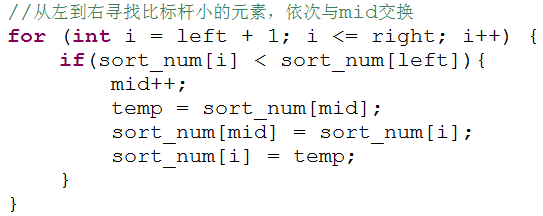3.最后一个比对元素跟标杆元素交换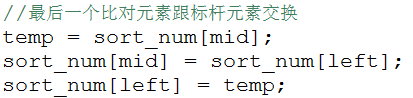4.比对结束，由中间结点划分两部分，递归重复1.2。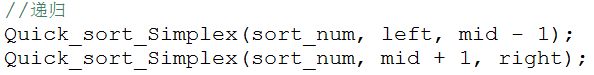单向快排注意事项
1）mid先加一，再交换                 2）为什么是left<right作为判定条件？                      因为递归调用quick_sort_duplexing(A, left, low-1)时，存在left>low-1使程序出错
双向快排设计思路
1.设高低指针，低指针从左往右找大于标杆的元素，高指针找小的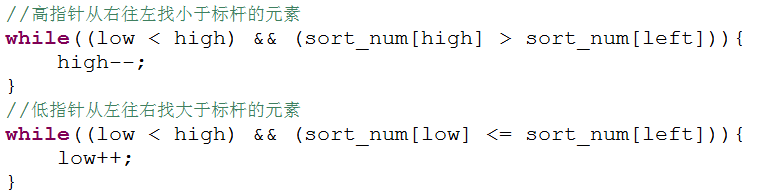2.交换高低指针所指向的元素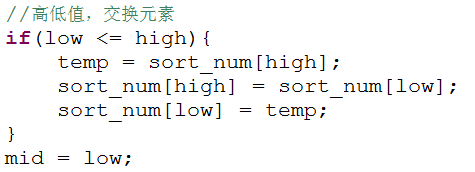3.交换标杆和低指针所指向的元素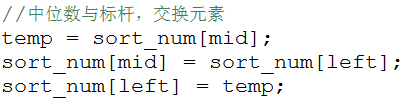4.依次递归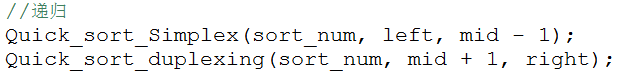双向快排注意事项
1）发生两次数值交换。                 2）left, right, low, high, mid,交换需注意。                 3）设计思路一中，高低指针与标杆元素对比时，必须有一个“=”号，不然陷入死循环。
快排改进
使用插入排序改进快排。
代码实现：
见我的github：快排及其优化
展开全文• 为避免此情况笔者编写了快排PRO 优化算法（也称三明治法）：将传统快排的二分法（小于中点值、大于等于中点值）更改为三分（小于中点值、等于中点值、大于中点值）。且对等于中点值部分不再递归，由此减少无效的排序...单链表 递归 算法
• 实验进行的是对快排使用插入排序进行优化，并对快排产生的不同的区间内元素个数值进行测试并计算运行时间，进行比较。代码已经进过测试可以使用
• 转载:百度快排,搜狗快排,360快排 以下转载github 上面文章 https://github.com/sopify-bot/seo 做个记录以便遗忘 背景： 我是2019年左右接触的seo这个事情，之前虽然混迹互联网圈子，也听过关于seo的事情，但是...seo
• 1、快速排序的基本思想： 快速排序使用分治的思想，通过一趟排序将待排序列分割成两部分，其中一部分记录的关键字均比另一部分记录的关键字小。之后分别对这两部分记录继续进行排序，以达到...(1)选择基准：在待
• SEO优化快排网站源码 信息流竞价营销类WordPress模板
• java快速排序，和随机优化快排 注解详细，多个版本可选，最简洁版、最高效率版、随机优化版...
• 对于快排而言，其核心在partition中，主要是对于pivot的选取上，所以我们可以按以下三种方案进行优化： 1.在数组长度大于某一个阈值范围时，我们进行递归快排，当数据长度小于阈值时，我们进行插入排序。 2.在...快速排序 partition
• 聚集相同元素排序是快速排序的一种优化方案，它的思路是在经过一次找基准之后把数据中与基准相同的数据聚集到基准左右，这样就可以少进行几次递归找基准的过程，从而提高了运行效率。 看以下程序： import java....算法
• 简介： 教程和源码已经打包了。需要的下载即可，很容易上手吗。 源码仅供技术参考，请于下载后24小时内删除，严禁商业用途！ 网盘下载地址： http://www.bytepan.com/pXCyWyGYw1t网站源码
• seo,百度快排,搜狗快排,360快排,百度排名优化； 百度快排,搜狗快排,360快排 背景： 我是2019年左右接触的seo这个事情，之前虽然混迹互联网圈子，也听过关于seo的事情，但是一直没什么动力，并没有什么实际性需求，...
• 快排的普通写法（递归） 时间复杂度（平均情况）：nlog2(n) int PartSort(int*arr,int first,int end) //分步排序函数 { int tmp=arr[first]; //取第一个数作为基准值 while(first!=end) { //取左边作为基准值...
• 到最后，整个序列就变得有序了 1、快速排序的方式(选取基准的方式)有三种： ① 固定位置选取基准法 ② 随机选取基准法 ③ 三分取中法 实现排序可以利用递归和非递归两种方式 2、快排优化： ① 当待排序序列中，...
• 基础快排：int __partition(int arr[], int l, int r) { int v = arr[l]; int j = l ; for (int i = l + 1; i &lt;= r; i++) { if (arr[i] &lt; v){ swap(arr[i], arr[j + 1]); j++; } } ...
• 然而我觉得博客还是要坚持日更，我相信时间总是挤出来的，不扯淡了，快排这是个面试常考题，今天主要着重于讲他的优化方法，那我就直接先贴快排代码，再来细细道来我所知道的优化方法，正常的快排，先上图片后上代码...算法 三向切分
• 快排，面试题中出现概率最高的一道，甚至没有之一。python实现，直接上代码def getMiddle(list,low,high): tmp = list[low] while(low ): while(low [high] > tmp): high -= 1 list[low] =递归 非递归实现
• 文章： 三种快排及四种优化方式 博文地址：https://blog.csdn.net/hacker00011000/article/details/52176100 1、快速排序的基本思想：  快速排序使用分治的思想，通过一趟排序将待排序列分割成两部分，其中一...快速排序
• 这篇文章是基于已经基本了解快排及其思想的人而设定的，不清楚快排基本思想和各种简单排序的同学可以先谷歌一下。 正文： 最简单的优化方法，基准值的选取。 基准值的选取影响到分治程度和递归深度，所以对快排来说...希尔排序 插入排序 数据库...